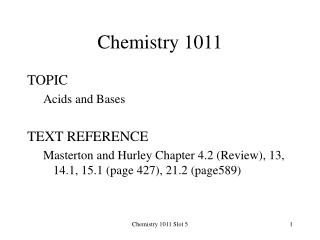DownloadDownload PresentationChemistry 1011

# Chemistry 1011

Télécharger la présentation## Chemistry 1011

- - - - - - - - - - - - - - - - - - - - - - - - - - - E N D - - - - - - - - - - - - - - - - - - - - - - - - - - -
##### Presentation Transcript

1. Chemistry 1011 TOPIC Acids and Bases TEXT REFERENCE Masterton and Hurley Chapter 4.2 (Review), 13, 14.1, 15.1 (page 427), 21.2 (page589) Chemistry 1011 Slot 5

2. 13.4 Weak Acids and Their Ionization Constants YOU ARE EXPECTED TO BE ABLE TO: • Distinguish strong acids and bases from weak acids and bases (Review Chapter 4) • Write an expression for the ionization constant, Ka, for a weak acid • Define pKa • Place a list of weak acids in order of strength given values for Ka or pKa • Calculate Ka, given the pH of a solution of a weak acid • Given Ka, calculate the percent ionization of a weak acid • Given Ka, calculate the [H+] and pH of a solution of a weak acid of known concentration Chemistry 1011 Slot 5

3. Strong and Weak Acids and Bases • Strong acids and bases are fully ionized in dilute aqueous solution HCl(aq) H+(aq) + Cl-(aq) NaOH(aq) Na+(aq) + OH-(aq) • Weak acids and bases are only partly ionized in dilute aqueous solution CH3COOH(aq) CH3COO-(aq) + H+(aq) Chemistry 1011 Slot 5

4. Strong and Weak Acids and Bases • Weak bases produce hydroxide ions by reacting with water molecules NH3(aq) + H2O(aq)NH4+(aq) + OH-(aq) cb ca ca cb • In this process, • the NH3 accepts a proton and is a Bronsted-Lowry base • the H2O donates a proton and is a Bronsted-Lowry acid • NH4+ is the conjugate acid of NH3 • OH- is the conjugate base of H2O Chemistry 1011 Slot 5

5. Ionization Constants of Weak Acids • Weak acids are only partly ionized in dilute aqueous solution CH3COOH(aq) CH3COO-(aq) + H+(aq) • This can also be written CH3COOH(aq) + H2O(aq)CH3COO-(aq) + H3O+(aq) acetic acid acetate ion Chemistry 1011 Slot 5

6. Ionization Constants of Weak Acids • The equilibrium constant can be written from either equation, ([H2O] is constant) Ka = [CH3COO-]x[H3O+] [CH3COOH] • In general, for HB(aq) H+(aq) + B-(aq) Ka = [H+]x[B-] [HB] Chemistry 1011 Slot 5

7. Ionization Constants of Weak Acids • The equilibrium constant, Ka, is known as the ionization constant • For acetic acid Ka = [CH3COO-]x[H3O+] = 1.8 x 10-5 [CH3COOH] • Note the low value: [CH3COO-] and [H3O+] will be small Chemistry 1011 Slot 5

8. pKa Values • The value of Ka can be used to compare the strength of different weak acids • Because of the very small values, it is sometimes useful to use pKa data for comparison pKa = -log10Ka Chemistry 1011 Slot 5

9. pKa for Some Weak Acids • ACID Ka pKa Nitrous acid, HNO2 6.0 x 10-4 3.22 Formic acid, HCOOH 1.9 x 10-4 3.72 Acetic acid, CH3COOH 1.8 x 10-5 4.74 Carbonic acid, H2CO3 4.2 x 10-7 6.38 Chemistry 1011 Slot 5

10. Calculating Ka from the pH Value CH3COOH(aq) CH3COO-(aq) + H+(aq) Ka = [CH3COO-]x[H+] [CH3COOH] • The pH of a 1.0 x 10-1 mol/L solution of acetic acid is 2.9. Calculate the value of Ka for the acid • (Ignore [H+] from the water - it is so small as to be insignificant) Chemistry 1011 Slot 5

11. Calculating Ka from the pH Value pH = -log10[H+] = 2.9 [H+] = 1.3 x 10-3 Ka = [CH3COO-]x[H+] = (1.3 x 10-3)x(1.3 x 10-3) [CH3COOH] (1.0 x 10-1 - 1.3 x 10-3) = (1.3 x 10-3)2 9.9 x 10-2 Ka = 1.7 x 10-5 Chemistry 1011 Slot 5

12. Percent Ionization • The % ionization of a weak acid HB is given by [H+](equilibrium) x 100% [HB](original) • For the acetic acid, the percent ionization is (1.3 x 10-3) x 100% = 1.3% (1.0 x 10-1) Chemistry 1011 Slot 5

13. Calculating [H+] and pH from Ka • Given that Ka for acetic acid is 1.8 x 10-5, calculate the pH of a 0.20mol/L solution. CH3COOH(aq) CH3COO-(aq) + H+(aq) [original] 2.0 x 10-1 0.0 0.0 D[ ] -x +x +x [equilibrium] (2.0 x 10-1- x) x x Ka = [CH3COO-]x[H+] = x2 [CH3COOH] (2.0 x 10-1- x) Chemistry 1011 Slot 5

14. Solving for x • If x is small compared to the initial concentration of the acid (% ionization is low), we can solve for x using an approximate method • If x is not small, the quadratic formula must be used • In this case, assume that x is small. Then (2.0 x 10-1 - x)  2.0 x 10-1 Chemistry 1011 Slot 5

15. Solving for x Ka = 1.8 x 10-5 = x2 (2.0 x 10-1) x2 = (1.8 x 10-5)(2.0 x 10-1) = 3.6 x 10-6 x = 1.9 x 10-3 mol/L pH = 2.7 (Check that [H+] is << [CH3COOH] ) 1.9 x 10-3 mol/L<< 2.0 x 10-1 mol/L Chemistry 1011 Slot 5

16. Solving for x Using the Quadratic Formula Ka = x2 = 1.8 x 10-5 (2.0 x 10-1 - x) x2 = (1.8 x 10-5) (2.0 x 10-1 - x) = (3.6 x 10-6) - (1.8 x 10-5 )x (x) x2 + (1.8 x 10-5 )x (x) - (3.6 x 10-6) = 0 • x = 1.9 x 10-3 mol/L • pH = 2.7 Chemistry 1011 Slot 5